# Weil cohomology

• ## General Introduction

This is the class of cohomology theories for which Pure motives (Chow motives) are supposed to have a universal property.

Examples include: Betti cohomology, Algebraic de Rham cohomology, Crystalline cohomology, l-adic cohomology, Etale cohomology (with finite coefficients), and I think also Rigid cohomology.

Weil cohomology theories are used to study Algebraic cycles. Some Weil cohomology theories are constructed using Sheaf cohomology

In the book project, the relevant chapter is the "Pure" chapter.

<]]>

<]]>
• ## Online References

A brief introduction by de Jong

Motivic stuff blog post

<]]>
• ## Paper References

Kleiman in Motives.

André: Une introduction aux motifs. Especially sections 3.3 and 3.4.

Grothendieck: The cohomology theory of abstract algebraic varieties. 1960 Proc. Internat. Congress Math. (Edinburgh, 1958) pp. 103--118 Cambridge Univ. Press

<]]>

<]]>
• ## Properties

See André for a presentation which differs slightly from Kleiman's below, for example in that André includes Tate twists in the discussion. André also reviews briefly the classical Weil cohomologies. Some notes:

A Weil cohomology is a (symmetric?) monoidal contravariant functor from smooth projective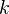-schemes to the tensor category of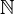-graded vector spaces (finite-dimensional in each degree), such that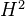of the projective line is one-dimensional. Also two additional requirements (1), (2) below.

Tate twist means tensoring with the inverse of the above cohomology space; not that it is invertible in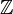-graded vector spaces because it is one-dimensional.

The cogebra structure on varieties, induced by the diagonal embedding, induces algebra structure on cohomology.

(1) Duality pairing, trace map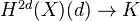(2) Cycle class map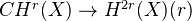André does not require weak or strong Lefschetz, because these statements lose their relevance when passing from pure to mixed.

A number of formal consequences, including: Various compatibilities, Gysin morphism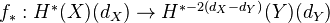(covariant!), projection formula, description of the trace map, relating Hom of cohomologies to cohomology of product, some stuff about the cycle map (and products, etc), removing of equidimensionality hyp through additivity, Lefschetz trace formula (trivial, from super-linear algebra).

Properties of homological equivalence.

Classical Weil cohomologies. In char zero: Etale cohomology with coeffs in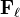,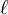-adic cohomology, algebraic de Rham cohomology, Betti cohomology, with their extra structures. In positive char:-adic cohomology, crystalline cohomology (whenis perfect).

Comparison IMs, including Tsuji and Berthelot-Ogus. Betti numbers.

Description of the various Tate twists, and of the part of cohomology which receives the cycle map.

Following Kleiman:

A Weil cohomology theory is a contravariant functor from the category of irreducible smooth projective varieties over an algebraically closed field, to the category of graded anticommutative algebras over a "coefficient field"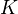of characteristic zero, such that the following holds.

1. (Finiteness) Each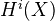has finite dimension, and vanishes unless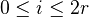, where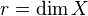.

2. (Poincaré duality) For each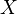of dimension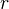, there is a funcorial "orientation" isomorphism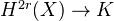which, when composed with the multiplication ("cup product") yields a non-degenerate bilinear pairing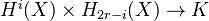. Notation: For a given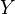of dimension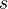and a map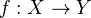, we write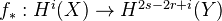for the transpose of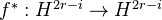.

3. (Kunneth) For all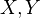, the projections induce an isomorphism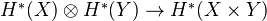.

4. (Cycle map) For each, write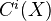for the group of algebraic cycles of codimension. Then there is a group HM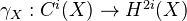satisfying:

• (Functoriality) For a map, have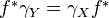and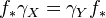• (Multiplicativity)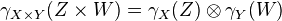• (Calibration) For a point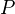,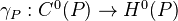is the canonical inclusion ofinto.
5. (Weak Lefschetz) Let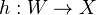be the inclusion of a smooth hyperplane section. Then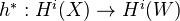is an isomorphism for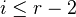and injective for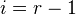.

6. (Strong Lefschetz)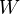as above. Define the Lefschetz operator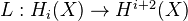by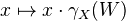. Then, for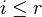,the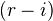'th iterate of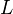is an isomorphism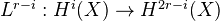.

<]]>
• ## Standard theorems

The cycle map preserves products.

If some nonzero multiple of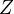is algebraically equivalent to zero, then the cycle map sendsto zero.

Write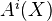for the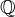-VS generated by the image of the cycle map in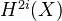.

We have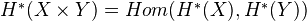. An element in this set is called a correspondence, and is called algebraic if it lies in.

Three important kinds of corresponndences: Graph, multiplication, and projector.

Thm: (Trace formula)

<]]>

<]]>

<]]>

<]]>

<]]>
• ## Some Research Articles

Lubkin: A p-adic proof of Weil's conjectures. Constructs a Weil cohomology for the cat in which an object is a-variety which lifts to a smooth proper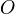-scheme. Hereis a complete discrete valuation ring with fraction field of char zero and residue field.

<]]>
• ## Other Information

There is an example of Serre about nonexistence of a cohomology theory with certain properties. Maybe this is related to this SBS post

http://mathoverflow.net/questions/27014/is-it-possible-to-classify-all-weil-cohomologies

<]]>